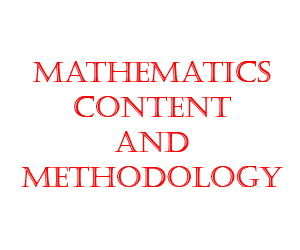# Mathematics TET Paper 1 Free Online Mock TestMathematics TET Paper 1 Free Online Mock Test

TRT TET Paper 1 MATHEMATICS

Mathematics mcq questions are very important objective multiple choice questions for crack TET Paper 1 in Telangana, Andhra Pradesh based on D.Ed, B.Ed books. This Mathematics Practice Test is very useful for students to get good score in TET, TRT examination.

Mathematics TET Paper 1 Free Online Mock Test is useful fro crack TS TET, AP TET, CTET, UP TET, KTET etcMathematics Syllabus For TET, TRT:
(Marks: 30)
CONTENT (Marks: 24)
1. Number System:
Natural Numbers, Whole Numbers, Integers, Rational Numbers & their fundamental
operations (addition, subtraction, multiplication and division). Primes Composite
Numbers, Co-Primes, Twin Primes, Relationship between LCM & GCD, Indian
currency, Representation of Natural, Whole, integers and rational numbers on a
number line. Terminating and Non-terminating decimals, Non-terminating but
recurring decimals, square, square root, cube, cube roots of numbers, Pythagorean
triplets. Applications on number system
2. Fractions:
Concept of Fractions, Proper Fractions, Improper Fractions, Mixed Fractions,
Decimal Fractions, Comparison, Fundamental operations on fractions (addition,
subtraction, multiplication and division), Representation of a fraction in pictorial form
and on a number line, reciprocal of fraction, uses of fractions in daily life
3. Arithmetic:
Unitary method, percentages, profit and loss, ratio and proportion, direct proportion,
discount, simple interest, compound interest, time and work, time and distance, tax
(Vat).
4. Geometry:
Basic idea of geometry (2D & 3D shapes), types of angles, construction and
measurement of angles, types of angles, lines, triangles, types of triangles,
triangles (SAS, SSS, ASA, RHS), construction of triangles and quadrilaterals,
patterns with geometrical shapes, representing 3D Shapes into 2D Shapes, Euler’s
relation, Properties of triangles, parallelogram, trapezium, Rhombus, Rectangle,
Square and Kite, Concept of Circles, Symmetry.
5. Measurements:
Length, Weight, Capacity, Time and their Standard Units, Surface Area and volume
of a cube and a cuboids, perimeter and area of triangle, quadrilateral, parallelogram,
rectangle, Rhombus, Square and Trapezium. Circumference of a circle, Area of
Circle, Circular paths, other polygons and sector in a circle.
6. Data Applications:
Introduction to Data, Data Presentation, Preparation of Frequency distribution table,
Bar Graph, Pictograph, Histogram, Mean, Median and Mode of ungrouped data,
determination of Mean by deviation method, Cumulative Frequency Distribution
Table, Frequency Polygon, Frequency Curve and Cumulative Frequency Curves.
7. Algebra:
Introduction to Algebra, Simple equations, solving linear equation in one variable,
exponents and powers, Algebric expressions, Addition, Subtraction, Multiplication,
Division and Factorisation of algebric expressions, algebric identities.

Mathematics PEDAGOGY (Marks: 06)
1. Definitions and Nature of Mathematics
2. Aims, values , instructional objectives of teaching Mathematics and Academic Standards
3. Methods of Teaching Mathematics
4. Instructional material in Mathematics – TLM in Mathematics
5. Instructional Planning
6. Continuous Comprehensive Evaluation (CCE) – Formative Assessment, Summative
Assessment – Processes and procedures
7. Designing, Administration, Analysis of scholastic Achievement test (SAT)
8. Diagnostic and Remedial Teaching
9. The Mathematics Teacher
10. Resource Utilization
11. Curriculum and Text Book

Total Questions:24
Marks: 24
Negative Marks: NO
Durations: 24 Minutes

## Leaderboard: TRT TET Paper 1 MATHEMATICS

maximum of 118 points
Pos. Name Entered on Points Result